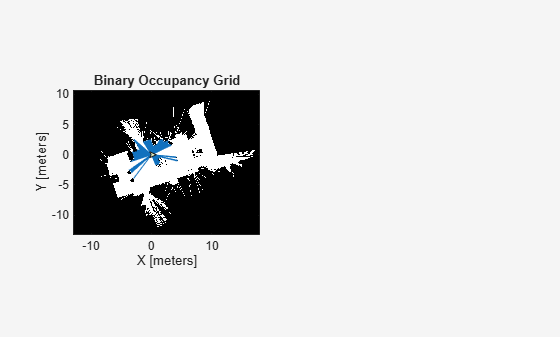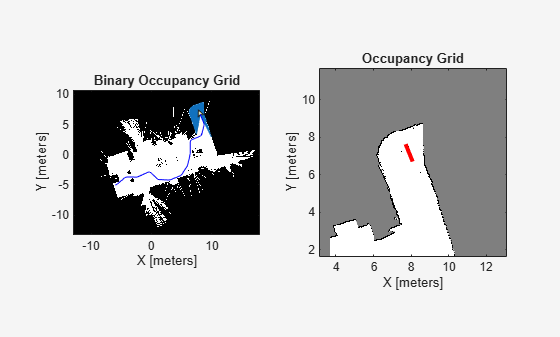# Create Egocentric Occupancy Maps Using Range Sensors

Occupancy Maps offer a simple yet robust way of representing an environment for robotic applications by mapping the continuous world-space to a discrete data structure. Individual grid cells can contain binary or probabilistic information, where 0 indicates free-space, and 1 indicates occupied space. You can build up this information over time using sensor measurements and efficiently store them in the map. This information is also useful for more advanced workflows, such as collision detection and path planning.

This example shows how to create an egocentric occupancy map, which keeps track of the immediate surroundings of the robot and can be efficiently moved around the environment. A trajectory is generated by planning a path in the environment and dictates the motion of the robot. As the robot moves around, the map is updated using sensor information from a simulated lidar and ground-truth map.

### Load a Prebuilt Ground-Truth Occupancy Map

Create a non-egocentric map from a previously generated data file, which is considered to be the ground truth for the simulated lidar. Load the map, `mapData`, which contains the `Data` field as a probabilistic matrix and convert it to binary values.

Create a `binaryOccupancyMap` object with the binary matrix and specify the resolution of the map.

```% Load saved map information load mapData_rayTracingTrajectory binaryMatrix = mapData.Data > 0.5; worldMap = binaryOccupancyMap(binaryMatrix,mapData.Resolution);```

Set the location of the bottom-left corner of the map, relative to the world origin.

```worldMap.LocalOriginInWorld = mapData.GridLocationInWorld; ```

Plot the ground truth. This example sets up a subplot for showing two maps side by side.

```set(gcf,'Visible','on') worldAx = subplot(1,2,1); worldHandle = show(worldMap,'Parent',worldAx); hold all```

### Create a Simulated Lidar

Create a `rangeSensor` object, which can be used to gather lidar readings from the simulation. You can modify various properties on the `rangeSensor` to more accurately represent a particular model of lidar, or add in sensor noise to test the robustness of your solution. For this example, set the `[min max]` range and the noise parameter. After creating the object, retrieve and plot a reading from the sensor by providing an `[x y theta]` pose relative to the world frame. Example helpers plot the robot, and the lidar readings overtop the worldMap.

```% Create rangeSensor lidar = rangeSensor; lidar.Range = [0 5]; lidar.RangeNoise = 0; pos = [0 0 0]; % Show lidar readings in world map [ranges, angles] = lidar(pos, worldMap); hSensorData = exampleHelperPlotLidar(worldAx, pos, ranges, angles); % Show robot in world map hRobot = exampleHelperPlotRobot(worldAx, pos);```### Initialize an Egocentric Map

Create an `occupancyMap` object to represent the egocentric map. Offset the grid-origin relative to the local-origin by setting the `GridOriginInLocal` property to half the difference between the world limits. This shifts the bounds of the map so that the local-origin is centered.

```% By default, GridOriginInLocal = [0 0] egoMap = occupancyMap(10,10,worldMap.Resolution); % Offset the GridOriginInLocal such that the "local frame" is located in the % center of the "map-window" egoMap.GridOriginInLocal = -[diff(egoMap.XWorldLimits) diff(egoMap.YWorldLimits)]/2;```

Plot the egocentric map next to the world map in the subplot.

```% Update local plot localAx = subplot(1,2,2); show(egoMap,'Parent',localAx); hold all localMapFig = plot(localAx,egoMap.LocalOriginInWorld+[0 1], egoMap.LocalOriginInWorld+[0 0],'r-','LineWidth',3);```

### Plan Path Between Points

We can now use our ground-truth map to plan a path between two free points. Create a copy of the world map and inflate it based on the robot size and desired clearance. This example uses a car-like robot, which has non-holonomic constraints, specified with a `stateSpaceDubins` object. This state space is used by the path planner for randomly sampling feasible states for the robot. Lastly, create a `validatorOccupancyMap` object, which uses the map to validate generated states, and the motions that connect them, by checking the corresponding cells for occupancy.

```% Copy the world map and inflate it. binaryMap = binaryOccupancyMap(worldMap); inflate(binaryMap, 0.1); % Create a state space object. stateSpace = stateSpaceDubins; % Reduce the turning radius to better fit the size of map and obstacle % density. stateSpace.MinTurningRadius = 0.5; % Create a state validator object. validator = validatorOccupancyMap(stateSpace); validator.Map = binaryMap; validator.ValidationDistance = 0.1;```

Use the RRT* planning algorithm, as a `plannerRRTStar` object and specify the state space and state validator as inputs. Specify start and end locations for the planner and generate a path.

```% Create our planner using the previously created StateSpace and StateValidator objects planner = plannerRRTStar(stateSpace, validator); planner.MaxConnectionDistance = 2; % Set a seed for the randomly generated path for reproducible results. rng(1, 'twister') % Set the start and end points. startPt = [-6 -5 0]; goalPt = [ 8 7 pi/2]; % Plan a path between start and goal points. path = plan(planner, startPt, goalPt); interpolate(path, size(path.States,1)*10); plot(worldAx, path.States(:,1),path.States(:,2),'b-');```

### Generate a Trajectory Along the Path

The planner generated a set of states, but to execute a trajectory, times for the states are needed. The goal of this example is to move the robot along the path with a constant linear velocity of `0.5` m/s. To get timestamps for each point, calculate the distances between points, sum them cumulatively, then divide this by the linear velocity to get a monotonically increasing array of timestamps, tStamps.

```% Get distance between each waypoint pt2ptDist = distance(stateSpace,path.States(1:end-1,:),path.States(2:end,:))```
```pt2ptDist = 139×1 0.1818 0.1818 0.1818 0.1818 0.1818 0.1818 0.1818 0.1818 0.1818 0.1818 ⋮ ```
```linVel = 0.5; % m/s tStamps = cumsum(pt2ptDist)/linVel;```

Generate a final simulated trajectory with `waypointTrajectory`, specifying the path states, the corresponding timestamps, and a desired sample rate of 10Hz.

`traj = waypointTrajectory(path.States, [0; tStamps], 'SampleRate', 10);`

### Simulate Sensor Readings and Build a Map

Lastly, move the robot along the trajectory while updating the map with the simulated Lidar readings.

To initialize the simulation, reset the trajectory, set the local origin to the first xy point on the trajectory, and clear the map.

```reset(traj); robotCurrentPose = path.States(1,:); move(egoMap, robotCurrentPose(1:2)); setOccupancy(egoMap,repmat(egoMap.DefaultValue,egoMap.GridSize));```

The primary operations of the simulation loop are:

• Get the next pose in the trajectory from traj and extract the z-axis orientation (theta) from the quaternion.

• Move the `egoMap` to the new [x y theta] pose.

• Retrieve sensor data from the lidar.

• Update the local map with sensor data using `insertRay`.

• Refresh the visualization.

```while ~isDone(traj) % Increment robot along trajectory [pts, quat] = step(traj); % Get orientation angle from quaternion rotMatrix = rotmat(quat,'point'); orientZ = rotm2eul(rotMatrix); % Move the robot to the new location robotCurrentPose = [pts(1:2) orientZ(1)]; move(egoMap, robotCurrentPose(1:2),'MoveType','Absolute'); % Retrieve sensor information from the lidar and insert it into the egoMap [ranges, angles] = lidar(robotCurrentPose, worldMap); insertRay(egoMap, robotCurrentPose,ranges,angles,lidar.Range(2)); % Update egoMap-centric plot show(egoMap, 'Parent', localAx, 'FastUpdate', 1); % Update orientation vector set(localMapFig, 'XData', robotCurrentPose(1)+[0 cos(robotCurrentPose(3))], 'YData', robotCurrentPose(2)+[0 sin(robotCurrentPose(3))]); % Update world plot exampleHelperUpdateRobotAndLidar(hRobot, hSensorData, robotCurrentPose, ranges, angles); % Call drawnow to push updates to the figure drawnow limitrate end```Note the robot drives through the environment, simulating sensor readings, and building an occupancy map as it goes.